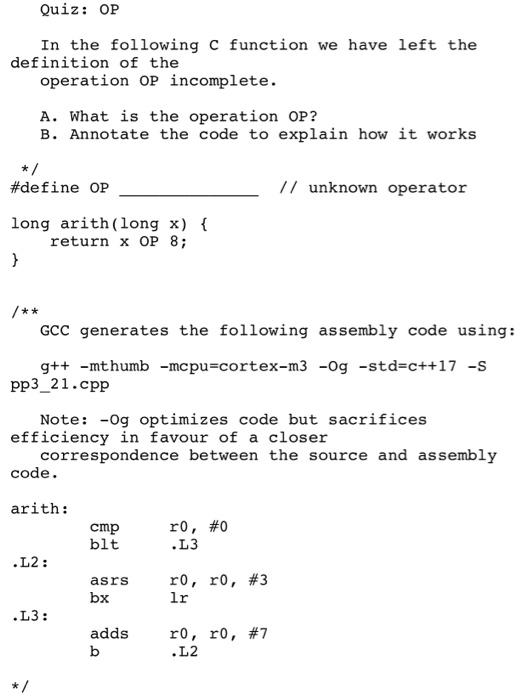# Quiz: OP In the following c function we have left the definition of the operation OP incomplete. A. What is the operation OP? B. Annotate the code to explain how it works #define OP // unknown operator long arith (long x) { return x OP 8; } /** GCC generates the following assembly code using: g++ -mthumb -mcpu-cortex-m3 -Og -std=c++17 -S pp3_21.cpp Note: -Og optimizes code but sacrifices efficiency in favour of a closer correspondence between the source and assembly code. arith: cmp blt r0, #0 .L3 .L2: asrs bx ro, ro, #3 lr .L3: adds b ro, ro, #7 .L2

Question-AnswerCategory: Computer ScienceQuiz: OP In the following c function we have left the definition of the operation OP incomplete. A. What is the operation OP? B. Annotate the code to explain how it works #define OP // unknown operator long arith (long x) { return x OP 8; } /** GCC generates the following assembly code using: g++ -mthumb -mcpu-cortex-m3 -Og -std=c++17 -S pp3_21.cpp Note: -Og optimizes code but sacrifices efficiency in favour of a closer correspondence between the source and assembly code. arith: cmp blt r0, #0 .L3 .L2: asrs bx ro, ro, #3 lr .L3: adds b ro, ro, #7 .L2

### Quiz: OP In the following c function we have left the definition of the operation OP incomplete. A. What is the operation OP? B. Annotate the code to explain how it works #define OP // unknown operator long arith (long x) { return x OP 8; } /** GCC generates the following assembly code using: g++ -mthumb -mcpu-cortex-m3 -Og -std=c++17 -S pp3_21.cpp Note: -Og optimizes code but sacrifices efficiency in favour of a closer correspondence between the source and assembly code. arith: cmp blt r0, #0 .L3 .L2: asrs bx ro, ro, #3 lr .L3: adds b ro, ro, #7 .L2Here OP is the division (/) operator .
because in assembly code
here in L2: there is comand asrs which is use as right shift operator for bit manupulation and
asrs r0 ,r0 ,#1 this means bits register r0 is shifted by one for ex. if r0=0111 ,after the command the r0=0011 (this means number is divided by to if we shift 1 bit)
similarly asrs r0 ,r0 ,#3 divides the number by 8 (shifting 3 bits)
and L3: part initialize the value of x to 7

``````long arith(long x)
{
return x / 8;
}``````

it will do 7/8 and return 0;
by using bits 7=(0111)2 and after right shifting 3 bits it become 0000